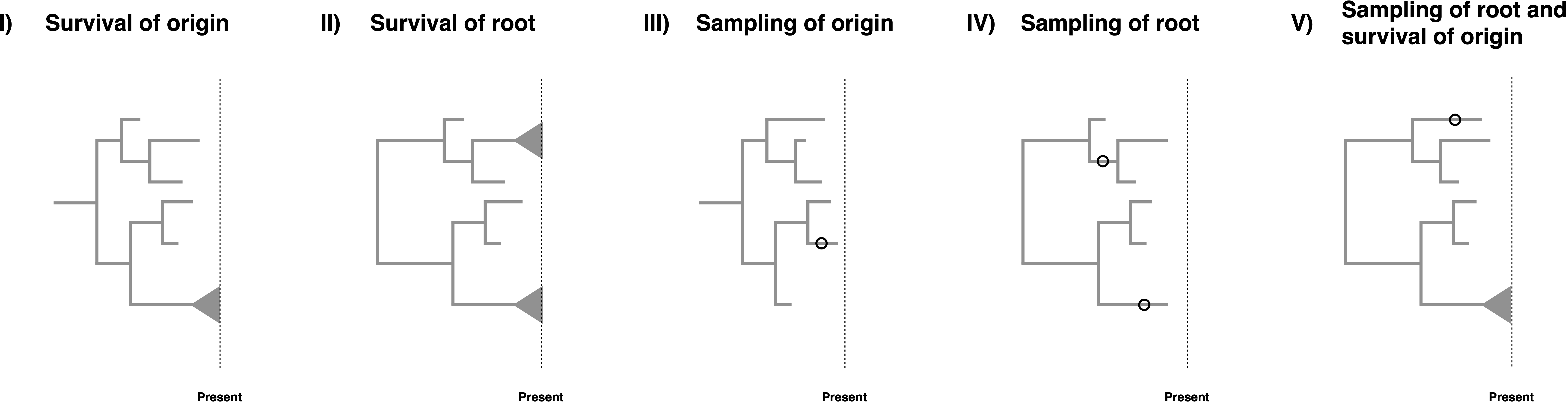# Assumptions of Diversification Rate Estimation

## Conditions of the Birth-Death ProcessFive different possible conditions for our generalized fossilized-birth-death process. I) The process survives until the present. II) The process starts at the root and both descendants of the root survive until the present. III) Sampling at least one lineage. IV) The process start at the root and both descendants have at least one lineage sampled. V) The process starts at the root, both descendants have at least one lineage sampled, and the process survives until the present.

Birth-death models are often conditioned on specific events, see (Stadler 2013), (Höhna 2015) and (Magee and Höhna 2021) for some discussion on the topic. However, when there are non-contemporaneous samples in the dataset which may be ancestral to other samples, conditioning becomes somewhat complex. The key issues for conditioning are whether it is assumed that the process starts at the root or the origin, and whether the descending lineage(s) is (are) assumed to leave any sampled descendant or specifically to have a descendant sampled at the present day. Consideration of these possibilities leads to five possible conditions, though conditioning is not strictly required.

• Survival of the origin: We condition the process on survival of one lineage, i.e., at least on descendant of the lineage starting at the origin was sampled at the present. This condition represent a case when we have fossils and extant taxa and do not know if the fossils are stem fossils of the entire clade. The condition is obtained by computing $1-E(t_{or})$ with $\phi(t)=0$.
• Survival of the root: We condition the process on survival of both lineages, i.e., at least one descendant of each lineage starting at the root was sampled at the present. This is the case for most macroevolutionary analyses without any fossils or if the fossils are known to belong within the crown group of the extant taxa. The condition is obtained by computing $(1-E(t_{MRCA}))^2$ with $\phi(t)=0$.
• Sampling of origin: We condition the process to have at least one sample being a descendant of the origin. This is simply a minimal condition that at least something was observed/sampled. This condition represents the case if we would also consider complete extinct clades. The condition is obtained by computing $1-E(t_{or})$.
• Sampling of the root: We condition the process to require that both lineages starting at the root are sampled. In this case, all taxa might be extinct but the root age is known or inferred as a parameter of the model. The condition is obtained by computing $(1-E(t_{MRCA}))^2$.
• Sampling of the root and survival of the origin: We condition the process on sampling of both descendant lineages of the root and at least one sample at the present. In this case, we condition on this specific root age but one of the descendant lineages of the root might have gone extinct while the other descendant lineage from the root must have survived. The condition is obtained by computing $(1-E_{\phi(t)=0}(t_{MRCA}))(1-E_{\phi(t)\neq 0}(t_{MRCA}))$.

For macroevolutionary analyses of diversification rates, condition (I) is the most adequate if we have both extinct and extant taxa, condition (II) if we have only extant taxa, and condition (III) if we have only extinct taxa. For phylodynamic applications, if it can safely be assumed that there are no sampled ancestors prior to the first observed infection (which will always be true if $r(t) = 1$), condition (IV) may be used, otherwise only condition (III) is applicable. Conditioning on survival as in (I), (II), or (V) requires $\Phi_0 > 0$, and so is primarily of interest in macroevolutionary applications. Of these conditions, (II) is the strictest and requires prior knowledge that the MRCA of the extant samples is the MRCA of all samples. Condition (V) is less restrictive, requiring only that none of the fossils could be sampled ancestors prior to the first observed speciation event, which would apply if all fossils are within the crown group. We could additionally condition on the number of extant taxa $N$, as suggest by (Gernhard 2008), although there is, as of today and to our knowledge, no solution known to condition on the number of extinct taxa.

1. Gernhard T. 2008. The conditioned reconstructed process. Journal of Theoretical Biology. 253:769–778. 10.1016/j.jtbi.2008.04.005
2. Höhna S. 2015. The time-dependent reconstructed evolutionary process with a key-role for mass-extinction events. Journal of Theoretical Biology. 380:321–331. http://dx.doi.org/10.1016/j.jtbi.2015.06.005
3. Magee A.F., Höhna S. 2021. Impact of K-Pg Mass Extinction Event on Crocodylomorpha Inferred from Phylogeny of Extinct and Extant Taxa. bioRxiv.:426715. 10.1101/426715
4. Stadler T. 2013. How can we improve accuracy of macroevolutionary rate estimates? Systematic Biology. 62:321–329.